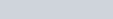ChemTalk

# Understanding Kinetic Molecular Theory### Core Concepts

You will learn the kinetic molecular theory of gases, and how to use the root-mean-square formula to determine the average speed of gas.

## What is the Kinetic Molecular Theory?

Kinetic molecular theory is a theory that explains the behavior of a gas in terms of the motion and interactions of its constituent particles, which are typically atoms or molecules. The theory has several key assumptions, including that the particles in a gas are constantly moving and colliding with one another, that the particles have no attractive or repulsive forces between them (except during collisions), and that the average kinetic energy of the particles is proportional to the temperature of the gas.

One of the key implications of kinetic molecular theory is that the motion of the gas particles determines the macroscopic properties of the gas, such as its pressure, temperature, and volume. For example, the pressure of a gas arises from the collisions of the gas particles with the walls of the container, while the temperature of a gas is directly related to the average kinetic energy of the particles.

Overall, the kinetic molecular theory provides a useful framework for understanding the behavior of gases and their interactions with other substances. It is an important concept in chemistry, physics, and engineering, and forms the basis for many important theories and models in these fields.

### Kinetic Molecular Theory – Key Points

• All gas particles move in random directions with random speeds.
• If particles are moving with equal probability in different directions, the average velocity will be 0.
• Recall that velocity is a vector, with both direction and magnitude, whereas speed is a scalar, with only magnitude.
• Average speed of gas particles can be calculated with the root-mean-square formula.

### Basic Assumptions

For kinetic molecular theory, we use the basic assumptions for ideal gases like helium, which are

• The volume occupied by a single gas particle is negligible in comparison to the volume occupied by the gas itself
• Gas particles exert no attractive or repulsive forces on other gas particles

We also must make assumptions regarding motion of particles, i.e.

• Gas particles are in motion and move randomly in one direction until they collide with another body
• Collisions between gas particles are completely elastic
• This means that when two gas particles collide, kinetic energy is conserved during the collision
• The kinetic energy of gas molecules is proportional to the absolute temperature only
• This means that as temperature decreases to absolute zero, particle kinetic energy and speed decrease to zero as well.

### What is Kinetic Molecular Theory?

So what is the kinetic molecular theory definition?

Kinetic molecular theory of gases is a way to describe the random motion of particles in a sample of gas. It states that all individual particles are moving in random directions with random speeds.

From our 5th assumption, recall that this random velocity is a function of absolute temperature. Because of this, you might assume that all particles would have the same speed. However, a sample of gas actually has a range of speeds, forming a normal distribution with a different height and width for different temperatures. By plotting these normal distributions together, we can see that as temperature increases, the amount of particles experiencing higher speeds increases, and if temperature decreases, the amount of particles experiencing higher speeds decreases.

In this graph, known as a Maxwell Botlzmann distribution,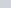represents the fraction of particles, and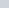represents the kinetic energy of the particle. The different lines represent normal distributions at different temperatures, in Celsius.

From this graph, it is clear that as temperature increases from -100 to 600, the amount of particles with higher kinetic energy increases.

### Effect of Particle Mass on Average Speed

Consider the equation for kinetic energy,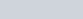From this equation, it is clear that for particles with the same kinetic energy, as mass increases, the velocity would decrease by a power of two. This can be shown by rearranging the equation to the form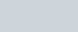By rearranging the equation, it is clear that velocity of a particle is proportional to the inverse square root of the mass of the particle.

### Root-Mean-Square Speed of Gas

We now know that temperature and mass both affect a gas’s average speed, so now we want an equation that relates both of these quantities to the average speed of a gas.

From kinetic molecular theory, we have the equation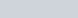By combining this equation with the equation relating velocity to kinetic energy, we can derive an equation relating mass and temperature of a particle to its velocity:Where M is the molar mass of the gas in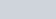,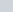is the temperature, and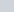is the universal gas constant.

From this equation, the average speed of a gas can be calculated.

### Kinetic Molecular Theory – Example Problem

What is the root-mean-square speed of a sample of Argon gas at 15 degrees Celsius?

Ans:
Molar mass of argon: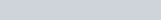Temperature in kelvin: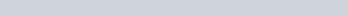Plugging in these values to the root mean square equation: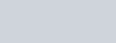## Kinetic Molecular Theory Practice Problems

Problem 1

You have a sample of three gases at the same temperature: Helium (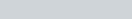), Argon (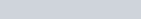), and Xenon (). Which gas has the highest average velocity?

Problem 2

An unknown gas sample has a root-mean-square speed of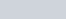. What is the gas’ molecular weight? (Bonus: what is the identity of the gas?)

## Kinetic Molecular Theory Practice Problem Solutions

1: Helium

2: